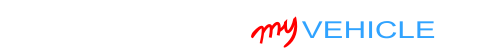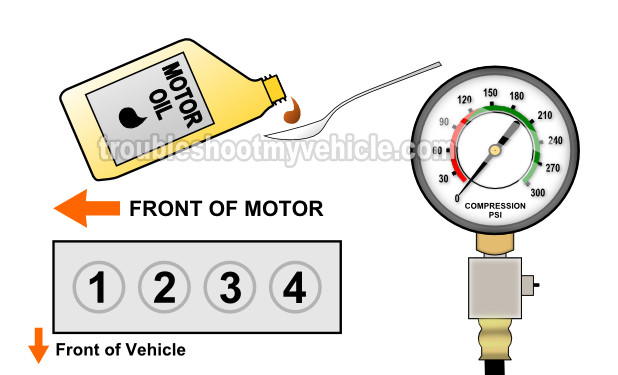## Interpreting The Results Of The Engine Compression Test

Depending on the amount of wear and tear and mileage on your mini-van's engine, the compression values of the cylinders will not be uniform.

Up to a certain point, this will not cause any engine performance problems. But if the values vary more than 15% between each other, then you'll have an engine performance problem.

Finding out if the compression values are within a 'good' range is not difficult and in this section I'll explain how to do it.

You can calculate this 15% difference with pen and paper or you can use my low compression calculator. You can find the low compression calculator here: Online Low Engine Compression Calculator (at: easyautodiagnostics.com).

If you want to manually calculate the 15% difference, here's what you'll need to do:

• STEP 1: Multiply the highest compression value by 0.15 (this is the decimal value of 15%).
• STEP 2: Round the result to the nearest one (for example: 25.6 would become 26).
• STEP 3: Subtract the result (the number that was rounded) from the highest compression value.
• ANSWER: The result of this subtraction is the lowest possible compression value any cylinder can have.

Now, let me give you a more specific example: Let's say that my engine compression test produced the following compression readings:

Cylinder Pressure
#1 165 PSI
#2   95 PSI
#3 155 PSI
#4 175 PSI

My next step is to do the following calculation:

• STEP 1:  175 x 0.15 = 26.25.
• STEP 2:  26.25 = 26 (rounded to nearest one).
• STEP 3:  175 - 26 = 149.
• ANSWER:  149 PSI. Any cylinder with this compression (or lower) value will misfire.

Since cylinder #2 is only producing 95 PSI, I can now conclude that it's 'dead' and causing a misfire.

To find out if the lowest compression value you got from your engine compression test is within a good range, you'll need to do the same calculation. Of course, you'll need to use the highest compression value you got and not the one in the example.

Once you've found the 'dead' cylinder, the next step is to find out what's causing the low compression value. For this step, go to: TEST 2: Wet Engine Compression Test.

## TEST 2: Wet Engine Compression TestIf in TEST 1 your compression test results indicate one or more cylinders will low or 0 PSI compression, then the next step is to perform a 'wet' compression test on them.

The 'wet' compression test simply involves adding about 2 tablespoons of engine oil to the cylinder with low compression and then retesting it with the compression gauge.

If the cylinder's compression value increases, then you can conclude that the compression problem is due to worn piston rings.

If the cylinder's compression value DOES NOT increase, then you can conclude that the compression problem is due to worn/damaged cylinder head valves.

Alright, lets get testing:

1. 1

Add a small amount of engine oil to the cylinder that reported low compression or no compression in the ‘dry’ compression test

The amount should be about 1 to 2 tablespoons of oil.

2. 2

Install the compression tester onto the cylinder.

Do not use any type of tool to tightened the compression tester. Hand tight is fine.

3. 3

When all is set up, have your helper crank the engine.

4. 4

You'll get one of two results:

1.) The compression value will go up (from the one you recorded before).

2.) The compression value will stay the same.

Let's take a look at what your test results mean:

CASE 1: The cylinder's compression value shot up. This confirms that the cylinder's low compression value is due to worn piston rings.

CASE 2: The cylinder's compression value stayed the same. This confirms that the cylinder's low compression value is due to worn or damaged cylinder head valves.If this info saved the day, buy me a beer!#### Latest

﻿

Chrysler Vehicles:

• Voyager 2.4L
• 2000, 2001, 2002, 2003

Dodge Vehicles:

• Caravan 2.4L
• 1996, 1997, 1998, 1999, 2000, 2001, 2002, 2003, 2004, 2005, 2006, 2007
• Grand Caravan 2.4L
• 1996, 1997

Plymouth Vehicles:

• Grand Voyager 2.4L
• 1996, 1997
• Voyager 2.4L
• 1996, 1997, 1998, 1999, 2000